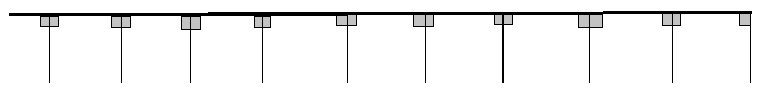# Math in Focus Grade 3 Chapter 17 Answer Key Angles and Lines

This handy Math in Focus Grade 3 Workbook Answer Key Chapter 17 Angles and Lines provides detailed solutions for the textbook questions.

## Math in Focus Grade 3 Chapter 17 Answer Key Angles and Lines

Math Journal

Question 1.
How would you explain perpendicular lines to a second-grader?
Two distinct lines intersecting each other at 90° or a right angle are called perpendicular lines.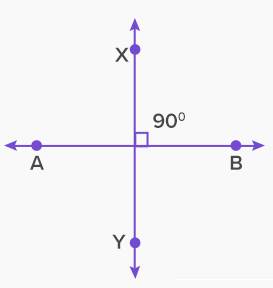Explanation:
Draw two straight lines,
which intersect each other.
Measure the angles of intersecting lines, it shows 90°.
So, Two distinct lines intersecting each other at 90° or a right angle are called perpendicular lines.
Question 2.
How would you explain parallel lines to a younger brother or sister?
First explain the concept of lines,
then explain them that the lines never meet each other are known ass parallel lines.
Example: Zebra crossing.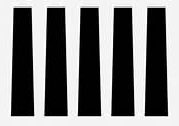Explanation:
Parallel lines can be defined as two lines in the same plane that are at equal distance from each other and never meet.

Question 3.
Lines that meet are perpendicular. Is this statement true? Use figures to explain your answer.
Explanation:A line that splits another line segment (or an angle) into two equal parts is called a “bisector.”
If the intersection between the two line segment is at a right angle, then the two lines are perpendicular,
and the bisector is called a “perpendicular bisector”.

Question 4.
Study the figure.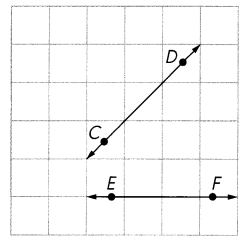Line segment CD is not perpendicular to line segment EF. How would you show that they are not parallel?
Explanation:
CD and EF are not perpendicular lines and,
if CD and EF are extended they will meet at one point C and E end one acute angle will form.

Challenging Practice

Draw each shape on the dot grid paper.

Question 1.
A shape with 5 sides and 5 angles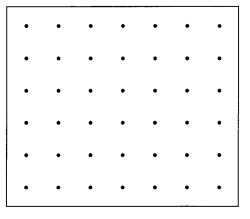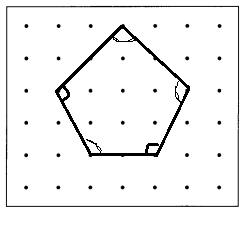Explanation:
5 sides and 5 angles is known as Pentagon.
A regular pentagon is a pentagon whose sides are equal in length, and whose interior angles are equal in measure.
Since each of the interior angles in a regular pentagon are equal in measure.

Question 2.
A shape with 3 sides and 2 angles less than a right angle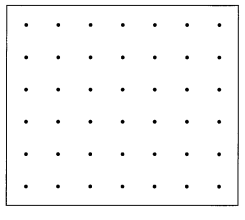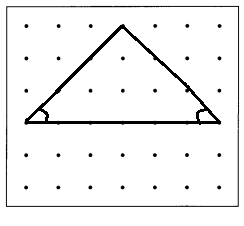Explanation:
A triangle is a type of polygon, which has three sides, and 2 angles are less than right angle.
The two sides are joined end to end is called the vertex of the triangle.
An angle is formed between two sides.

Question 3.
A shape with 4 sides and an angle greater than a right angle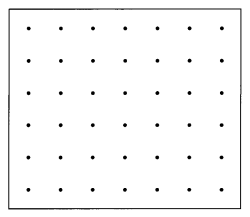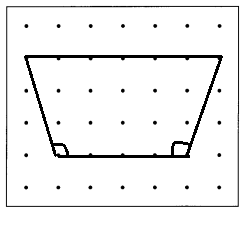Explanation:
4 sides and an angle greater than a right angle
Isosceles trapezoid one pair of opposite sides are parallel and the base angles are equal in measure.
Alternative definitions are a quadrilateral with an axis of symmetry bisecting one pair of opposite sides,
or a trapezoid with diagonals of equal length.

Question 4.
A shape with 7 sides and 7 angles, 3 of which are right angles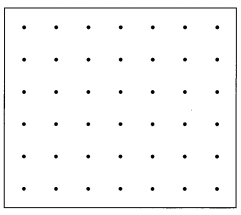7 sides and 7 angles, 3 of which are right angles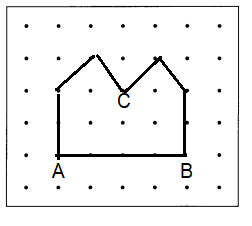Explanation:
A heptagon is a polygon that has seven sides.
It is a closed figure having 7 vertices.
A heptagon is also sometimes called Septagon.
In Geometry, the shape that is bounded by at least three straight lines or at least three interior angles is called polygon.

Question 5.
Name two pairs of perpendicular line segments.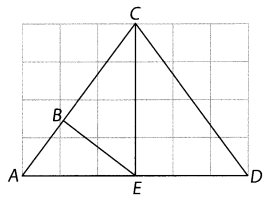AC and BE perpendicular line segments and
AD and EC perpendicular line segments.
Explanation:
The intersection between the two line segment is at a right angle, then the two lines are perpendicular.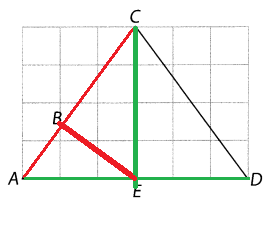Question 6.
Name four line segments parallel to segment NM.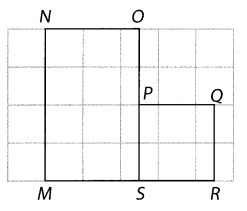OS, OP, PS, QR are four line segments parallel to segment NM.
Explanation:
four line segments parallel to segment NM are shown below;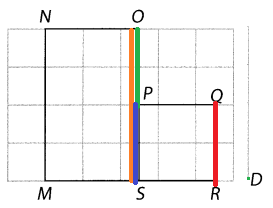OS, PS , OP and QR  are parallel lines to NM
Two lines, line segments, or rays are said to be parallel,
if they never meet and are always the same distance apart.

Question 7.
How many pairs of parallel line segments are in the diagram?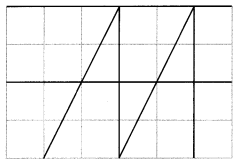There are ____________ pairs of parallel line segments.
There are  3 pairs of parallel line segments.
Explanation:
The below picture shows 3 pairs of parallel line segments with 3 different colors.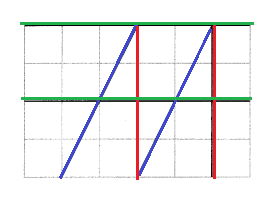Two lines, line segments, or rays are said to be parallel,
if they never meet and are always the same distance apart.

Problem Solving

Draw shapes on dot grid paper. Then answer the questions.

Question 1.
Draw a triangle with a right angle.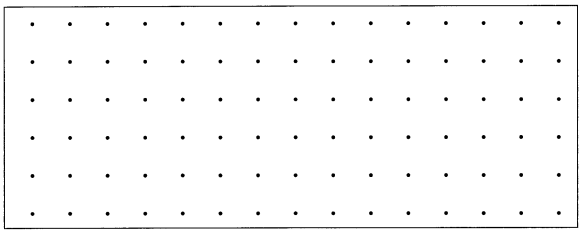Can you draw a triangle with 2 right angles? __________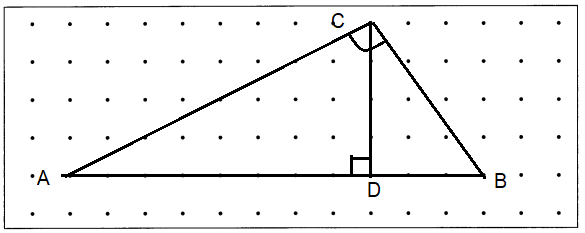Explanation:
2 right angles, ∠CDA and ∠CDB
one right angle at C and one right angle at D.

Question 2.
Draw a shape with 4 sides and 2 right angles.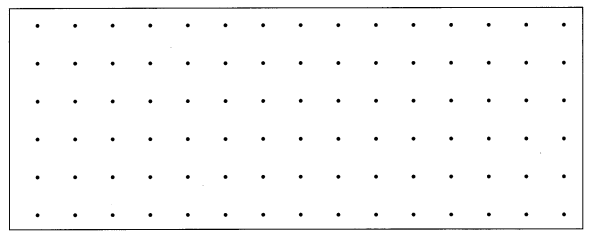What do you notice about the other angles in the shape?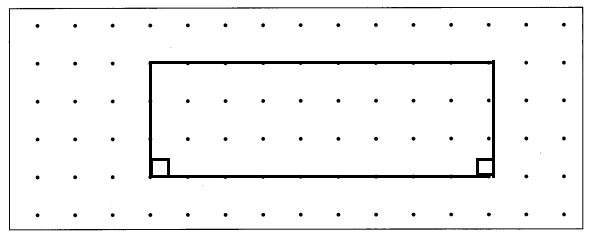Explanation:
The shape is rectangle with 4 sides and 2 right angles.
It is a type of quadrilateral that has its parallel sides equal to each other and all the four vertices are equal to 90 degrees. Hence, it is also called an equiangular quadrilateral.
Solve.

Question 3.
Five students are walking to school from their houses. Look at the map.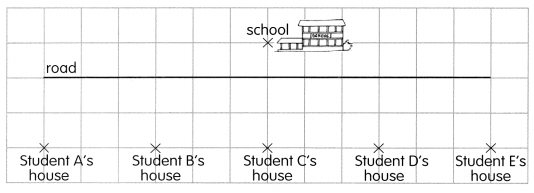Which student’s house is nearest to the school? Student ___________ Draw a line segment to join this student’s house to the school. What do you notice about this line segment?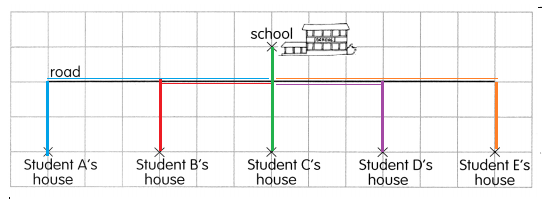Explanation:
From the above given information:
Student’s house is nearest to the school is C.
A line segment is bounded by two distinct points on a line.
Or we can say a line segment is part of the line that connects two points.
A line has no endpoints and extends infinitely in both the direction but a line segment has two fixed or definite endpoints.

Question 4.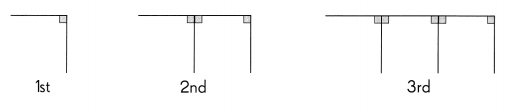The pattern is made up of perpendicular line segments. The 1st pattern has 1 right angle. The 2nd pattern has 3 right angles. The 3rd pattern has 5 right angles. How many right angles will the 10th pattern have?